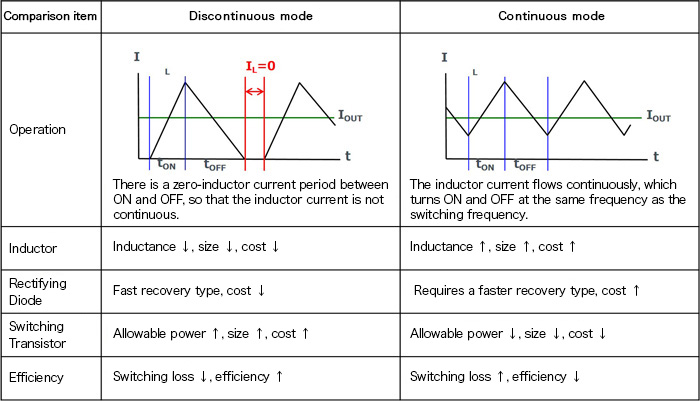# What are Buck Converters? – Basic Operation and Discontinuous Mode vs. Continuous Mode

2017.09.07

・Step-down converter operation is in either a continuous mode or a discontinuous mode.

・In DC-DC conversion, the continuous mode is generally used, but in AC-DC conversion at about 60 W, the discontinuous mode is often used.

In our discussion of non-isolated AC-DC converter design, we begin by explaining circuit operation. The AC-DC converter we use as an example is generally called a “buck” converter. Originally a buck converter meant a step-down converter, but the term came to be used for DC-DC converters as well. While there are various theories, conventional standard step-down converters were diode-rectified (asynchronous) devices, and it became customary to refer to diode-rectified step-down converters as buck converters. Regardless of the names used, there are a number of step-down methods used in step-down converters, and the step-down converter of this example is the previously mentioned diode-rectified device.

## Operation of Buck Converters

Below, a model of a basic step-down converter is used to explain the circuit operation. By gaining an understanding of the properties of current pathways and nodes from the basic operation, standards for selection of peripheral components and matters demanding attention will become clear. In the diagrams, we replace the high-side transistor and low-side diode with switches to explain operation schematically. The circuit principles are the same as those of diode rectification in a DC-DC converter, but the high voltage obtained by rectifying an AC voltage is directly switched to perform step-down voltage conversion, and so the transistor and diode acting as switches must withstand high voltages, for example 600 V or so.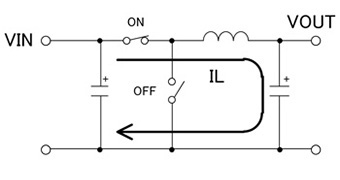• ・When the high-side switch (the transistor) turns on, a current IL flows in the inductor L, and energy is stored
• ・At this time, the low-side switch (the diode) is turned off
• ・The inductor current IL is expressed by the following equation （ton： ON-time）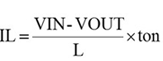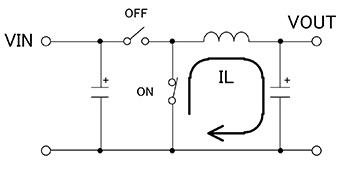• ・When the high-side switch (the transistor) turns off, the energy stored in the inductor is output through the low-side switch (the diode)
• ・At this time, the high-side switch (the transistor) is OFF
• ・The inductor current IL is expressed by the following equation （toff： OFF time）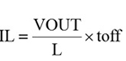## Discontinuous Mode and Continuous Mode

In switching operation, there are two modes, a discontinuous mode and a continuous mode. They are compared in the following table.

The “operation” item for comparison is the waveform of the currents flowing in the primary windings and secondary windings of the transformer. In discontinuous mode, there is a period in which the inductor current IL is interrupted, hence the name, discontinuous mode. In contrast, in continuous mode there is no period in which the inductor current is zero.

In each mode, arrows indicate the tendencies for the inductor, the rectifying diode, the switching transistor, and the efficiency; an upward arrow “↑” means an increase, and a downward arrow “↓” indicates a decrease.

In the case of the continuous mode, when the switches are ON, a reverse current flows during the reverse recovery time (trr) of the rectifying diode, and losses occur due to this reverse current. In low-voltage switching DC-DC conversion, the reverse voltage of the rectifying diode is low and the reverse current is also small, and so generally the continuous mode is used, giving priority to reducing the output ripple voltage and harmonics. However, in AC-DC conversion, the diode reverse voltage is high and a large reverse current flows, and so discontinuous mode, in which a reverse current does not flow and losses are reduced, is generally used. However, the peak current becomes large, and when the load is large, sometimes operation in continuous mode is preferred.

Each mode has its advantages and disadvantages, but in general, the discontinuous mode is used up to about 50 to 60 W. At output powers above this, a decision is made taking into account the size of the transformer that can be accommodated and other factors. In this design example, the discontinuous mode is used.